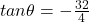## Three friends tie three ropes in a knot and pull on the ropes in different directions. Adrienne (rope 1) exerts a 20-N force in the positive

Question

Three friends tie three ropes in a knot and pull on the ropes in different directions. Adrienne (rope 1) exerts a 20-N force in the positive x-direction, and Jim (rope 2) exerts a 40-N force at an angle 53∘ above the negative x-axis. Luis (rope 3) exerts a force that balances the first two so that the knot does not move.
a. Use equilibrium conditions to find F LonKx and F LonKy.
b. Use equilibrium conditions to determine the magnitude of F⃗ LonK.
c. Use equilibrium conditions to determine the angle θ that describes the direction of F⃗ LonK. Use positive values if the force is directed above the positive x-axis and negative values if it is directed below the positive x-axis.
d. Construct a force diagram for the knot.

in progress 0
3 weeks 2021-08-23T23:02:02+00:00 1 Answers 0 views 0

Part a)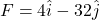Part b)

Magnitude of the force is given as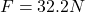Part c)

As we know that Y component of the force is negative so here the force is directed below the X axis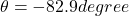Explanation:

Part a)

Adrienne apply the force of 20 N along +X direction and Jim apply force of 40 N at 53 degree above negative X axis

so we will have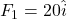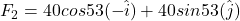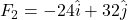now let say the force exerted by Luis is F such that sum of all forces must be zero

now we have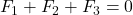so we have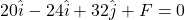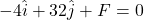Part b)

Magnitude of the force is given as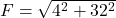Part c)

As we know that Y component of the force is negative so here the force is directed below the X axis

So here we have